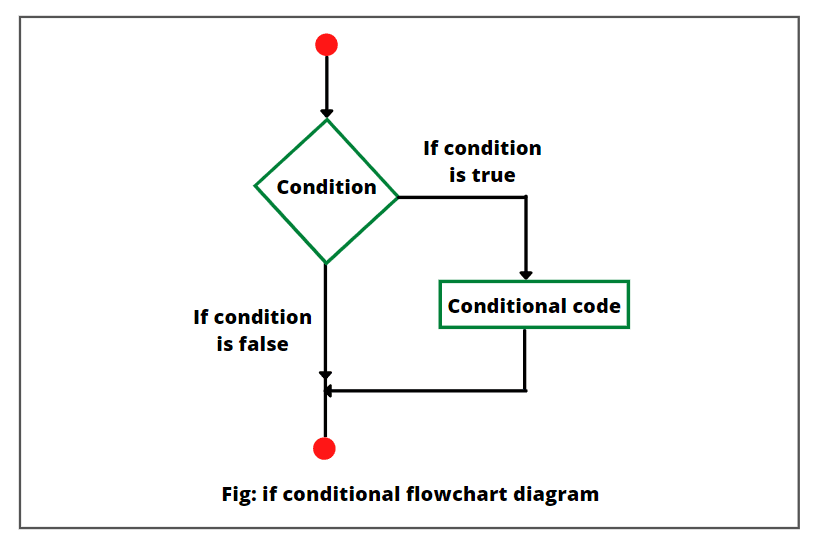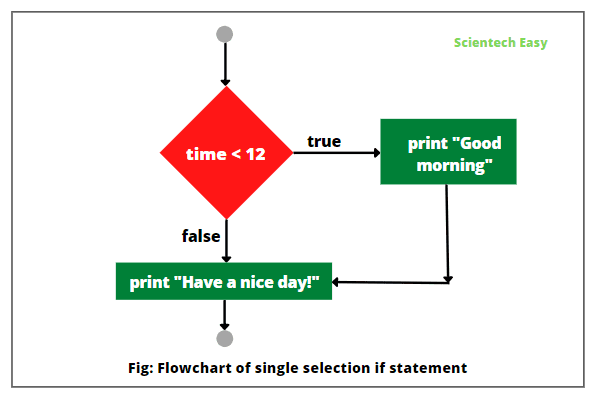# If Statement in JavaScript | Example Program

An if statement in JavaScript is the simplest decision-making statement that allows to specify an alternative path of execution in a program. It is used to change the flow of execution in the program.

This statement executes a block of statements when the condition is true, and skips them if the condition is false.

If statement is also called conditional control statement or single selection statement in JavaScript because it selects or ignores a single action or a single group of actions.

## Syntax of if Statement

If statement in JavaScript consists of a boolean expression followed by one or more statements. The general syntax for using the if statement is as follows:

```if(Test condition)
{
JavaScript statement(s) to be executed if condition is true.
}
```

In the above expression,

a) The condition consists of a boolean expression that returns a boolean value, either true or false.

b) The test condition contains any relational comparison consisted of relational operators and must be enclosed within parentheses.

c) If the test condition is true, the statement executes. If the test condition is false, the statement skips or bypass.

d) If the block of if statement (also called body of if statement) contains more than one statement, must enclose these statements within a pair of braces ({ }).

## Flowchart Diagram of If statement in JavaScript

Look at the below figure, where you can see the if statement flowchart diagram.Let’s understand the single selection if statement with the help of an example. Consider the following program code as given below.

```let myPer = 92;
if(myPer >= 80)
```

If the boolean expression (condition) evaluates to true, the statement will execute and print the message “Grade A” on the browser.

After executing the statement inside the block of if statement, the next JavaScript statement in the sequence executes.

If the boolean expression evaluates to false, the statement will not execute and the next statement in the sequence executes. Let’s understand a very simple example.

```if(true)
document.write("Code to be executed"); // print.

if(false)
document.write("Code not to be executed"); // not print.
```

Similarly, consider the following script code.

```if (radius >= 0)
{
document.write("Area of circle: " +area);
}
```

If the boolean expression evaluates to true, statements in the block of if statement executes. In other words, if the value of radius is greater than or equal to 0, the area of circle will be computed, and the result will be displayed on the browser.

Otherwise, the two statements in the block will be skipped, not executed, and continue with the rest of program.

Let’s create a JavaScript program in which we will implement the following example code.

Program code 1:

```<script>
pi = 3.14;
{
document.write("Area of circle: " +area);
}
</script>
```
```Output:
Area of circle: 12.56
```

In the above code, if the condition in the if statement evaluates true, statements inside the block of if statement will execute. Otherwise, statements inside block will not execute if the condition evaluates false.

Some Valid if statements in JavaScript are as follows:

```1. let x = 1;
if(x > 0)
document.write(x+ " is a positive number");

2. let x = 1;
if(x) // same as: if(x != 0)
document.write(x+ " is a nonzero number");

3. let x = 10, y = 10;
if(x == y)
document.write("x and y are equal number");

4. let x = 5, y = 10;
if(x < y) {
document.write("x is less than y");
}
5. let x = true;
if(x) // Here, we have used a boolean value to check whether the condition is true or not.
document.write("You are eligible for voting");
```

## Types of Selection Statements in JavaScript

JavaScript supports many types of selection statements that are as follows:

• One way if statements
• Two-way if-else statements
• Nested if statements
• Multi-way if-else statements
• Switch statements
• Conditional expressions

We will explore all types of selection statements one by one in detail in the further tutorial.

## Example Program based on If Statement in JavaScript

Let’s take some example programs based on a single selection if statement in JavaScript.

1. Let’s create a JavaScript program in which we will prompt marks for three subjects, such as math, chemistry, and physics from the user.

Then we will calculate the percentage of three subject marks and display “Grade A” if the percentage is greater than or equal to 85. Look at the script code to understand better.

Program code 2:

```<script>
let phy, chem, math;
// Prompt the user to enter marks of three subjects.
phy = prompt("Enter your physics marks: ");
let p = parseInt(phy);
chem = prompt("Enter your chemistry marks: ");
let c = parseInt(chem);
math = prompt("Enter your math marks: ");
let m = parseInt(math);

let totalMarks = p + c + m;
let myPer = totalMarks/3;
document.write("Total marks obtained: " +totalMarks, "<br>");
if(myPer >= 85.0) // if the condition is true, then the statement will be displayed.
</script>
```
```Output:
Total marks obtained: 273
```

When you run the above program, the browser will display a dialog box to prompt the user to enter marks of three subjects. When you will enter marks in the dialog box, it will return them in the string form.

So, we need to convert string into integer. For this, we have used the parseInt() method that converts string into integer.

2. Let’s create a JavaScript program for a web application to prompt the user to enter a number. If the number is divisible by 2, the program displays a message “number is divisible by 2”.

Program code 3:

```<script>
// Prompt the user to a number.
let num = prompt("Enter a number: ");
if(num % 2 == 0)
document.write(num+ " is divisible by 2.");

if(num % 2 != 0)
document.write(num+ " is not divisible by 2.");
</script>
```
```Output:
35 is not divisible by 2.
10 is divisible by 2.
```

3. Let’s write a JavaScript code in which we will display a “Good morning” greeting if the time on the browser is less than 12. If the time on the browser is greater than 12, it will display “Have a nice day!” on the browser.

Program code 4:

```<script>
var date = new Date();
var time = date.getHours();
if(time < 12)
{
document.write("<B>Good morning</B>");
}
document.write("<b>Have a nice day!</b>");
</script>
```
```Output:
Have a nice day!
```

While writing this program, the time on the browser is greater than 12 o’clock, therefore, JavaScript interpreter evaluates false and does not execute the block of if statement.

Then, the control of execution jumps to the next statement in the program. Look at the flow chart diagram below:## Use of Logical Operators in If Statement in JavaScript

JavaScript logical operators can also be used in the conditional expression when we need to check multiple conditions together. There are three types of logical operators in JavaScript that are as follows.

• && (AND)
• || (OR)
• ! (NOT)

Let’s see some example programs based on logical operators used in the if statement.

Program code 5: Logical AND (&&) operator

```<script>
let x = 20, y = 40, z = 50;
if((y > x) && (y < z)) // true
document.write("y is greater than x but smaller than z", "<br>");

if((x > y) && (y < z)) // false
document.write("z is greater than x, y", "<br>");

if(y % x == 0 && x != 0) // true
document.write("y is divisible by x");
</script>
```
```Output:
y is greater than x but smaller than z
y is divisible by x
```

In the preceding example program, the first, and third if statements evaluates true as both conditional expression joined by && operator are true.

The second if statement evaluates false, as the first conditional expression is false. In this example, if statement will produce true only if both expressions are true.

If any of the expressions is false or both expressions are false, if statement will produce false.

Program code 6: Logical OR (||) operator

```<script>
let x = 2, y = 1, z = 4;
let value;
if(value = (x > y) || (y < z))
document.write(value, "<br>");

if(value = (x > y) || (y > z))
document.write(value, "<br>");

if(value = (x < y) || (y < z))
document.write(value, "<br>");

if(value = (x < y) || (y > z))
document.write(value);
</script>
```
```Output:
true
true
true
```

In the above example program, if any of the expressions are true or both expressions are true, if statement evaluates true. If both expressions are false, the if statement evaluates false and the block of statements does not execute.

Program code 8: Logical NOT (!) operator

```<script>
let x = 2, y = 1;
let value;
if(value = (x == 2) && (y != 2))
document.write(value);
</script>
```
```Output:
true
```

We can combine several conditional expressions by using “Logical Not” operator. The result will be a boolean type.

In this tutorial, you have learned a single selection if statement with various example programs. Hope that you will have understood all the basic points and concepts of if statement.

In the next tutorial, we will understand if-else statement with various example programs.